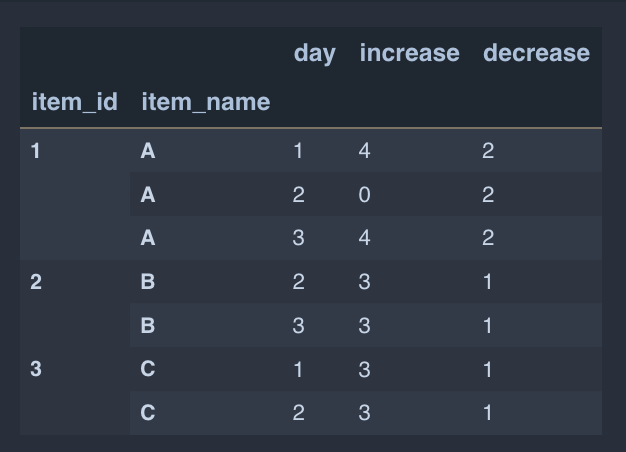# How to set each first unique multi-index to 0 and calculate values for the others

Based on the following sample data, the following data frame is built:

```day = [1, 2, 3, 2, 3, 1, 2]
item_id = [1, 1, 1, 2, 2, 3, 3]
item_name = ['A', 'A', 'A', 'B', 'B', 'C', 'C']
increase = [4, 0, 4, 3, 3, 3, 3]
decrease = [2, 2, 2, 1, 1, 1, 1]
my_df = pd.DataFrame(list(zip(day, item_id, item_name, increase, decrease)),
columns=['day', 'item_id', 'item_name', 'increase', 'decrease'])
my_df = my_df.set_index(['item_id', 'item_name'])```I would like to create two new columns:

1. starting_quantity would have each initial value set to 0 for the index (or multi-index)
2. ending_quantity adds the `increase` and subtracts the `decrease`
3. starting_quantity[1, 2, 3, …] is equal to the ending_quantity of the previous day.

The output I’d like to create is as follows:I appreciate if you could assist with any or all of the 3 steps above!

Contents

### Method 1

Try:

``````my_df = my_df.set_index(["item_id", "item_name"])
g = my_df.groupby(level=0)

my_df["tmp"] = my_df["increase"] - my_df["decrease"]

my_df["starting_quantity"] = g["tmp"].shift().fillna(0)
my_df["starting_quantity"] = g["starting_quantity"].cumsum().astype(int)

my_df["ending_quantity"] = g["tmp"].cumsum()
my_df = my_df.drop(columns="tmp")

print(my_df)
``````

Prints:

``````                   day  increase  decrease  starting_quantity  ending_quantity
item_id item_name
1       A            1         4         2                  0                2
A            2         0         2                  2                0
A            3         4         2                  0                2
2       B            2         3         1                  0                2
B            3         3         1                  2                4
3       C            1         3         1                  0                2
C            2         3         1                  2                4
``````